# RS Aggarwal Class 9 Solutions Chapter 14 - Statistics

## RS Aggarwal Class 9 Chapter 14 - Statistics Solutions Free PDF

It is a branch of mathematics that deals with the collection of statistical data which is used for a variety of different mathematical techniques especially in the field of linear algebra, differential equations and measure theory. The tools used in Statistics are observational studies and natural experiments, which are an important resource for the collection of data. Statistics collects data in the form of surveys using random sampling. The data analysis of the statistical data can be divided into:

1. Inferential statistics
2. Descriptive statistics

Learn more about RS Aggarwal Class 9 Solutions Chapter 14 Statistics, available below:

## Statistics Solutions RS Aggarwal Chapter 14

Q.1: Define statistics as a subject.

Sol:

Statistics is a branch of science which deals with the collection, presentation, analysis, and interpretation of numerical data.

Q.2: Define some fundamentals characteristics of statistics.

Sol:

Fundamental characteristics of statistics:

(i) It deals with the numerical data.

(ii) The qualitative characteristic such as illiteracy, intelligence, poverty etc. cannot be measured numerically.

(iii) Statistical inference is not exact.

Q.3: What are primary data and secondary data? Which of the two is more reliable and why?

Sol:

Primary data: Primary data is the data collected by the investigator himself with a definite plan in his mind. These data are very accurate and reliable as these being collected by the investigator himself.

Secondary data: Secondary data is then collected by a person other than the investigator.

Secondary data is not very reliable as these are collected by others with the purpose other than the investigator and may not be fully relevant to the investigation.

Q.4: Explain the meaning of each of the following terms:

Sol:

(i) Variant: Any character which can assume many different values is called a variant.

(ii) Class Interval: Each group or class in which data is condensed is called a class interval.

(iii) Class-Size: The difference between the true upper limit and the true lower limit of a class is called class size.

(iv) Class-mark: The average of upper and lower limit of a class interval is called its class mark. i.e. Class mark= Upperlimit+lowerlimit2$\frac{Upper \; limit \; + lower \; limit }{2}$

(v) Class limit: Class limits are the two figures by which class is bounded. The figure on the left side of a class is called lower limit and on the right side is called its upper limit.

(vi) True class limits: In the case of an exclusive form of frequency distribution, the upper-class limits and the lower class limits are the true upper limits and the lower limits. But in the case of an inclusive form of frequency distribution, the true lower limit of a class is obtained by subtracting 0.5 from the lower limit of the class. And the true upper limit of the class is obtained by adding 0.5 to the upper limit.

(vii) The frequency of a class: The number of observations falling in a class determines its frequency.

(viii) Cumulative frequency of a class: The sum of all frequencies up to and including that class is called the cumulative frequency of that class.

Q.5: Following data gives the number of children in 40 families:

1,2,6,5,1,5,1,3,2,6,2,3,4,2,0,4,4,3,2,2,0,0,1,2,2,4,3,2,1,0,5,1,2,4,3,4,1,6,2,2.

Represent it in the form of frequency distribution, taking classes 0-2, 1-2, etc.

Sol:

Minimum observation is 0 and maximum observation is 6. The classes of equal size covering the given data are: (0 – 2), (2 – 4), (4 – 6) and (6 – 8).

Thus, the frequency distribution may be given as under: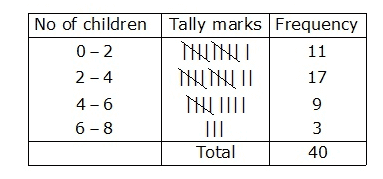Q.6: The marks obtained by 40 students of a class in an examination are given below:

3, 20, 13, 1, 21, 13, 3, 23, 16, 13, 18, 12, 5, 12, 5, 24, 9, 2, 7, 18, 20, 3, 10, 12, 7, 18, 20, 3, 10, 12, 7, 1, 8, 2, 5, 7, 10, 16, 8, 16, 17, 8, 23, 24, 6, 23, 15

Present the data in the form of a frequency distribution using equal class size, one such class being 10 – 15 (15 not included)

Sol:

Minimum observation is 1 and maximum observation is 24. The classes of equal size from the given data are:

(0-5), (5-10), (10-15), (15- 20), (20-25)

Thus, the frequency distribution may be given as under: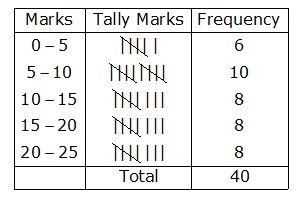Q.7: Construct a frequency table for the following ages(in years) of 30 students using equal class intervals, one of them being 9-12, where 12 is not included.

18, 12, 7,6,11,15,21,9,8,13,15,17,22,19,14,21,23,8,12,17,15,6,18,23,22,16,9,21,11, 16

Sol:

Minimum observation is 6 and maximum observation is 23. So the range is 23-6 = 17

The classes of equal size covering the given data are: (6-9), (9-12), (12-15), (15-18),(18-21), (21-24).

Thus the frequency distribution may be given as under: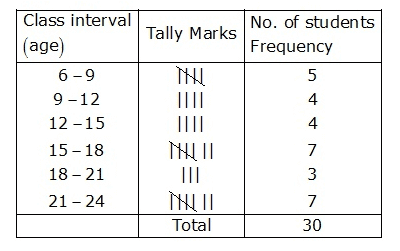Q.8: Construct a frequency table with equal class intervals from the following data on the monthly wages (in rupees) of 28 laborers working in factory, taking one of the class intervals as 210-230 (230 not included): 220, 268, 258, 242, 210, 268, 272, 242, 311, 290, 300, 320, 319, 304, 302, 318, 306, 292, 254, 278, 210, 240, 280, 316, 306, 215, 256, 236.

Sol:

Minimum observation is 210 and maximum observation = 320

So the range is (320-210) =110

The classes of equal size covering the given data are:

(210-230), (230-250), (250-270), (270-290), (290-310), (310-330)

Thus the frequency distribution may be given as: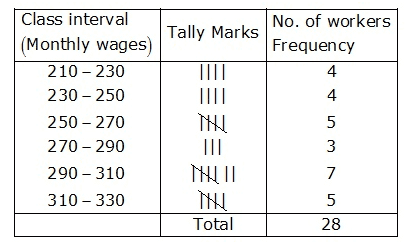Q.9: The weight (in grams) of 40 oranges picked at random from a basket is as follows:

40, 50, 60, 65, 45, 55, 30, 90, 75, 85, 70, 85, 70, 85, 75, 80, 100, 110, 70, 55, 30, 35, 45, 70, 80, 85, 95, 70, 60, 70, 75, 40, 100, 65, 60, 40, 100, 75, 110, 30, 45, 84. Construct a frequency table as well as a cumulative frequency table.

Sol:

Minimum observation is 30 and maximum observation is 110.

So the range is 100-30 =80

The classes of equal size covering the given data are:

(30-40), (40-50), (50-60), (60-70), (70-80), (80-90), (90-100), (100-110), (110-120)

Thus the frequency and cumulative frequency table may be given as under: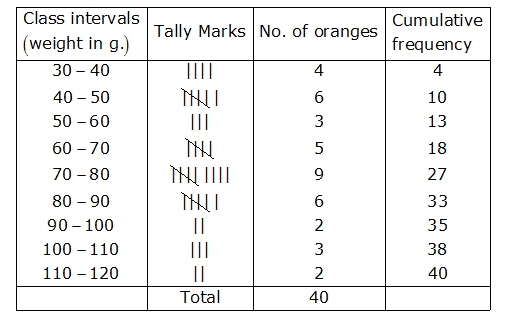Q.10: The weekly wages (in rupees) of 30 workers in factory are given below: 830, 835, 890, 810, 835, 836, 869, 845, 898, 890, 820, 860, 832, 833, 855, 845, 804, 808, 812, 840, 885, 835, 836, 878, 840, 868, 890, 806, 840, 890.

Represent the data in the form of a frequency distribution with class size 10.

Sol:

Minimum observation is 804 and maximum observation is 898.

So, range is 898 – 804 = 94

The classes of equal covering the given data are:

(800-810), (810-820), (820-830), (830-840), (840-850), (850-860), (860-870), (870-880), (880-890), (890-900).

Thus the frequency table may be given as: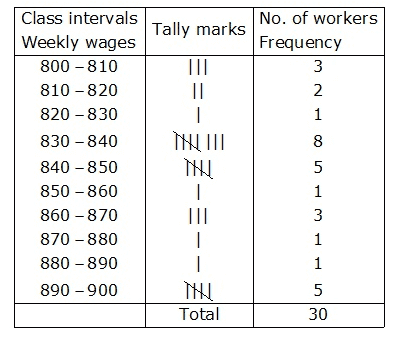Q.11: The electricity bills (in rupees) of 40 houses in a locality are given below:

116, 127, 107, 100, 80, 82, 91, 101, 65, 95, 87, 81, 105, 129, 92, 75, 89, 78, 87, 81, 59, 52, 65, 101, 115, 108, 95, 65, 98, 62, 84, 76, 63, 128, 121, 61, 118, 108, 116, 130.

Construct a grouped frequency table.

Sol:

Minimum observation is 52 and maximum observation is 130

So, the range is 130-52= 78

The class of equal size covering the given data is:

(50-60), (60-70), (70-80), (80-90), (90-100), (100-110), (110-120), (120-130), (130-140)

Thus, the frequency table may be given as: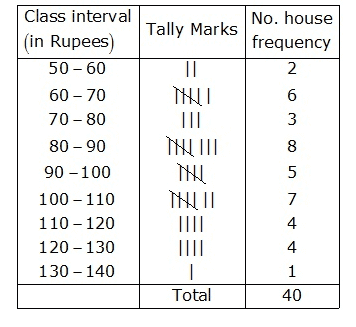Q.12: Following are the ages (in years) of 360 patients, getting medical treatment in a hospital:

 Age(in years) 10-20 20-30 30-40 40-50 50-60 60-70 No. of patients 90 50 60 80 50 30

Construct the cumulative frequency table for the above data

Sol:

 Age (in years) Number of Patients (Frequency) Cumulative Frequency 10-20 90 90 20-30 50 140 30-40 60 200 40-50 80 280 50-60 50 330 60-70 30 360 Total 360 360

Q.13: Present the following as an ordinary grouped frequency table:

 Marks(below) 10 20 30 40 50 60 No. of students 5 12 32 40 45 48

Sol:

 Marks Number of students (Cumulative Frequency) Class Intervals Frequency 10 5 0-10 5 20 12 10-20 12-5=7 30 32 20-30 32-12=20 40 40 30-40 40-32=8 50 45 40-50 45-40=5 60 48 50-60 48-45=3 Total 48

Q.14: Given below is cumulative frequency table:

 Marks No. of students Below 10 17 Below 20 22 Below 30 29 Below 40 37 Below 50 50 Below 60 60

Extract a frequency table from the above.

Sol:

 Marks (below) Number of students (Cumulative Frequency) Class Intervals Frequency 10 17 0-10 17 20 22 10-20 22-17=5 30 29 20-30 29-22=7 40 37 30-40 37-29=8 50 50 40-50 50-37=13 60 60 50-60 60-50=10 Total 60

Q.15: Make a frequency table from the following:

 Marks obtained No. of students More than 60 0 More than 50 16 More than 40 40 More than 30 75 More than 20 87 More than 10 92 More than 0 100

Sol:

 Marks (below) Number of students (Cumulative Frequency) Class Intervals Frequency More than 60 0 More than 60 0 More than 50 16 50-60 16-0=16 More than 40 40 40-50 40-16=24 More than 30 75 30-40 75-40=35 More than 20 87 20-30 87-75=12 More than 10 92 10-20 92-87=5 More than 0 100 0-20 100-92=8 Total 100